Want to share your content on R-bloggers? click here if you have a blog, or here if you don't.

Earlier this week, I published a post about song lyrics and how different U.S. states are mentioned at different rates, and at different rates relative to their populations. That was a very fun post to work on, but you can tell from that paragraph near the end that I am a little bothered by the uncertainty involved in calculating the rates by just dividing two numbers. David Robinson suggested on Twitter that I might try using empirical Bayes methods to estimate the rates. I am a newcomer to Bayesian methods but this idea makes a lot of sense in this context, so let’s see what we can do!

## Getting Started

The analysis here borrows very heavily from two of Dave’s posts from last year. To start out, what are the values that we are dealing with? (I have hidden the code that downloads/calculates these values, but you can see the entire R Markdown file here.)

We have, for each state here, the population in the state, the number of times it was mentioned in a song, and the rate of mentions per population (just the previous two numbers divided by each other). The reason I was uncomfortable here is that some states were mentioned so few times (like Hawaii and Montana!) and it is surely true that the rates calculated here have very different uncertainty intervals from state to state.

## Bayesian Who?!

This is where Bayesian methods come in. We can use Bayesian methods to a) estimate the rate itself and b) estimate credible intervals. For a really wonderful explanation of how Bayesian models work, I will point you to Rasmus Bååth’s post about whether his wife is pregnant or not. He posted that last year not too long after Dave posted some of his baseball/Bayes posts, at which time I started to think, “Maybe this Bayes stuff does actually make sense.” To use a Bayesian method, we need to choose a prior probability distribution for what we want to estimate; this is what we believe about the quantity before evidence is taken into account. What makes empirical Bayes “empirical” is that the prior probability distribution is taken from the data itself; we will plot the actual distribution of rates (mentions per population) and use that distribution as our prior.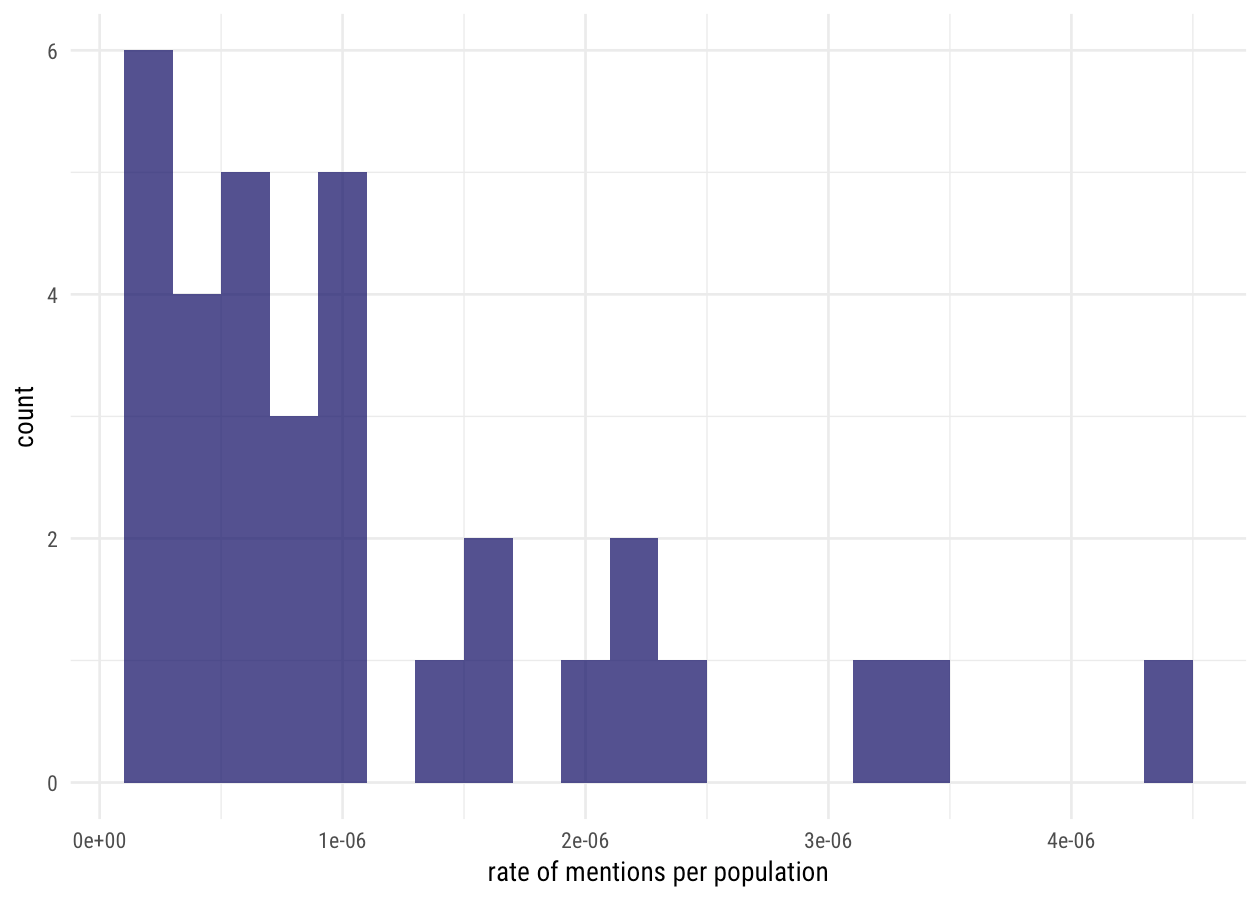Hmmmmm, that’s not so great, is it? There are only 50 states and not all of them were mentioned in this lyrics data set at all. But we will merrily push forward and calculate a prior probability distribution from this. Let’s fit a beta distribution to this to use as our prior. I’m going to use the method of moments to fit a beta distribution.

Now let’s plot this.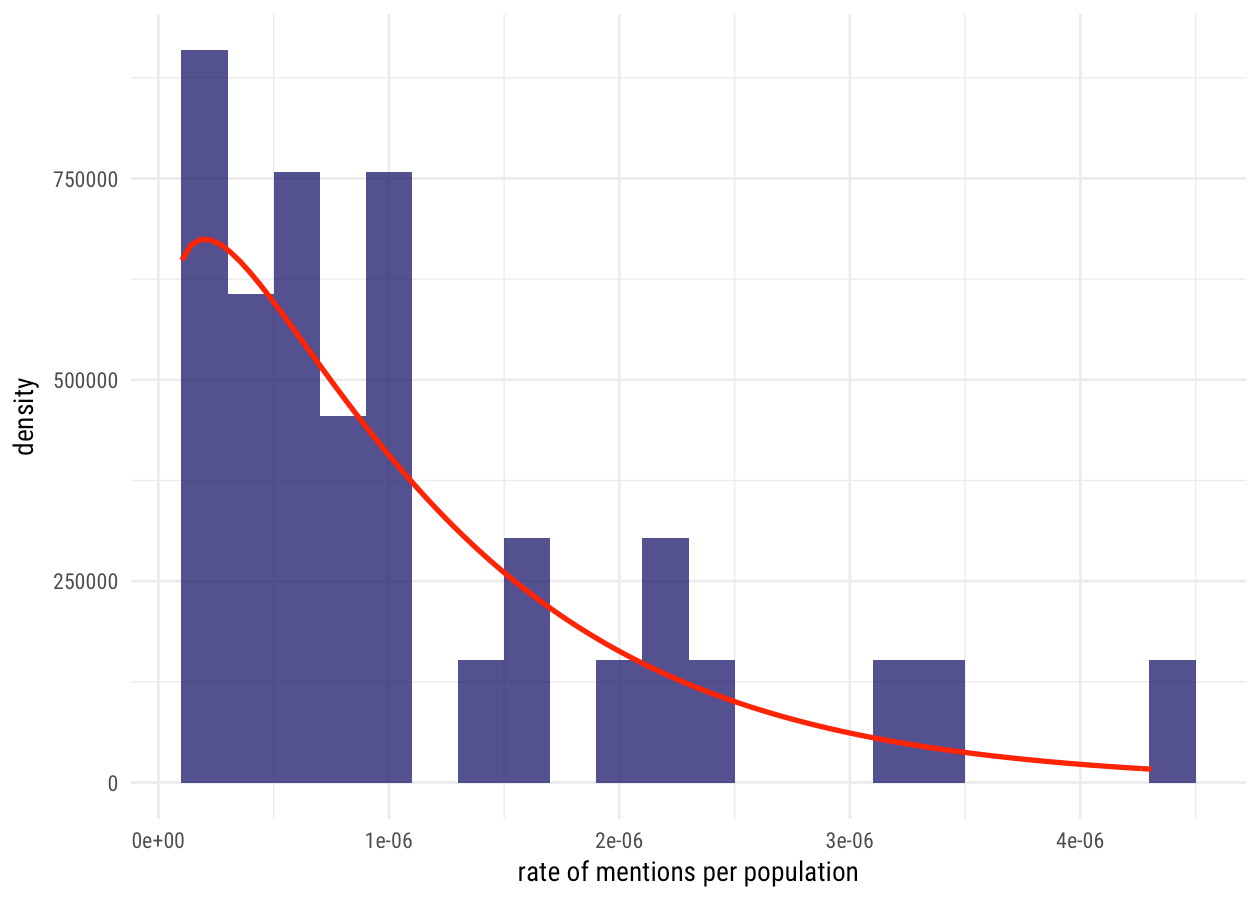That’s not… too awful, I hope. Remember, what this is supposed to be is our belief about the distribution of rates before evidence from individual states is taken into account. I would buy this in a general sense; there a few states that are mentioned many times and many states that are mentioned a few times.

And that’s it! We have a prior.

## Calculating the Empirical Bayes Estimate

Now that we have a prior probability distribution, we use Bayes’ theorem to calculate a posterior estimate for each state’s rate. It’s not very difficult math.

How do the two values compare?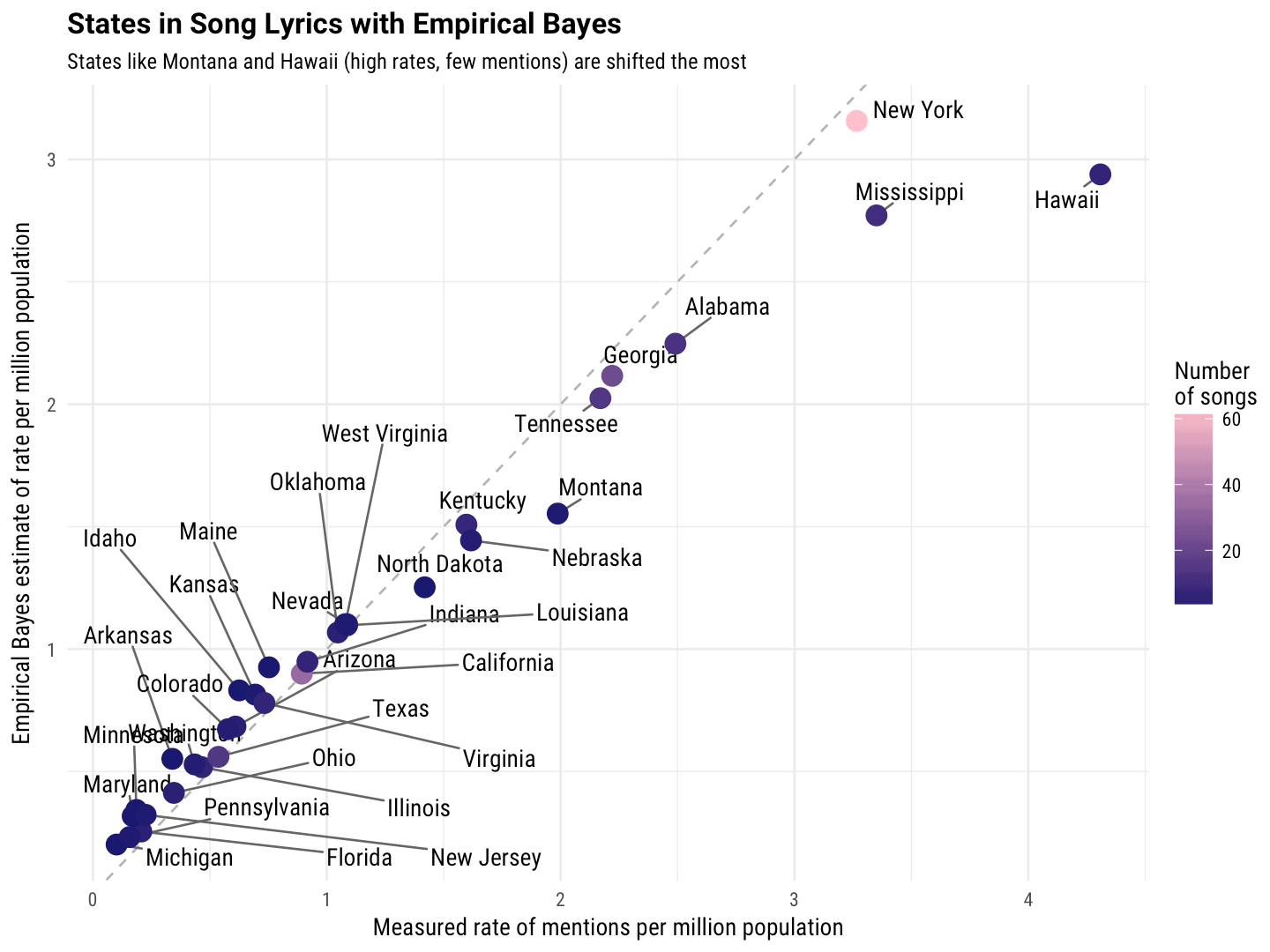Notice that the states that were mentioned the highest number of times are closest to the line, i.e., the empirical Bayes method here did not change the value that much. It is only for states that were mentioned in a few songs that the two values are quite different. Notice that the high rates were shifted to lower values and the low rates were shifted to (slightly) higher values.

## What Is the Posterior Distribution for Each State?

We calculated an empirical Bayes estimate for each rate above, but we can actually calculate the full posterior probability distribution for each state. What are $\alpha$ and $\beta$ for each state?

Let’s plot a few of these to see what they look like.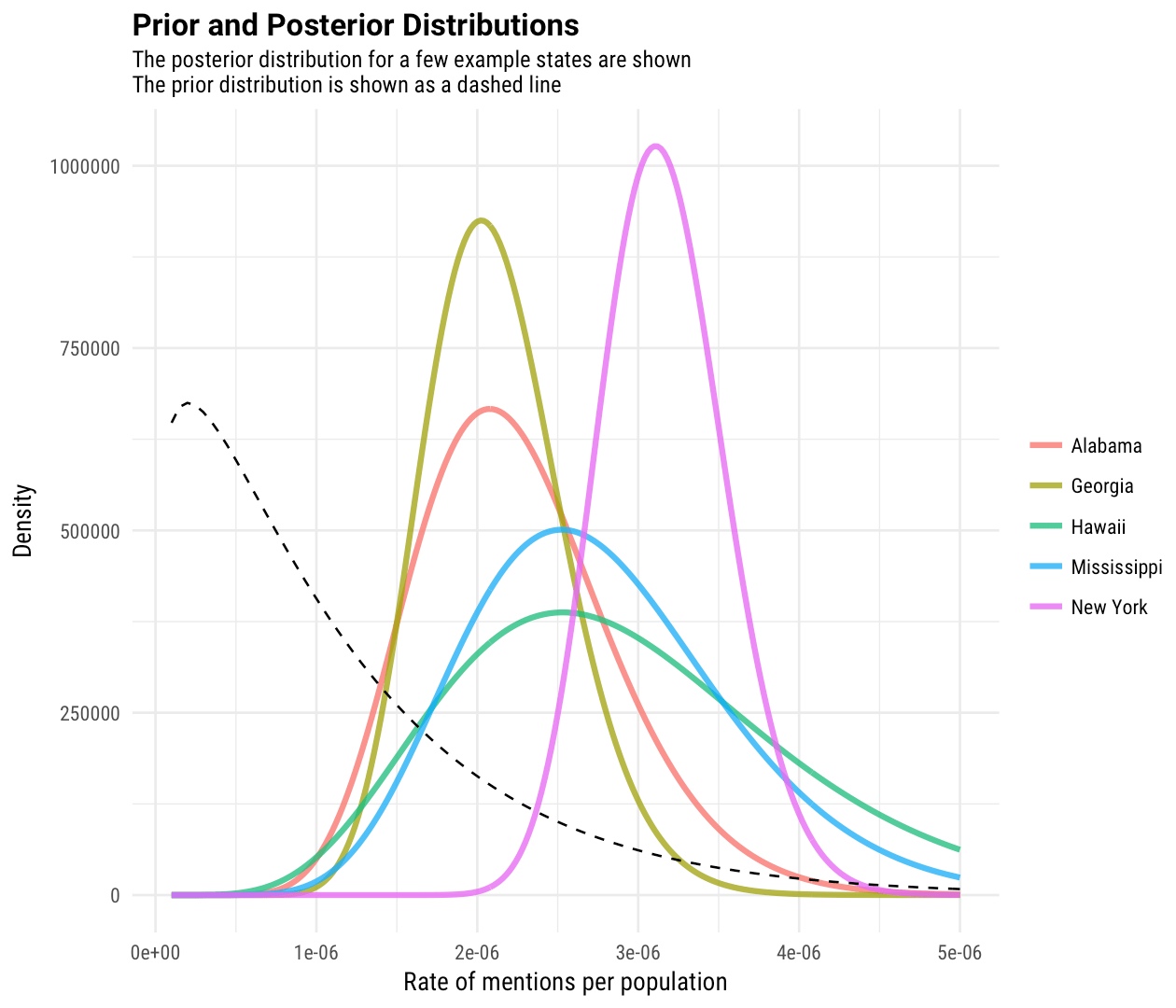Notice that New York, which was mentioned in many songs, has a narrow posterior probability distribution; we have more precise knowledge about the rate for New York. Hawaii’s posterior probability distribution is centered at about the same spot but it is a broad probability distribution; we have less precise knowledge about the rate for Hawaii, because Hawaii was only mentioned in a few songs!

We can use these posterior probability distributions to calculate credible intervals, an estimate of how uncertain each of these measurements is, analogous to a confidence interval. (BUT SO DIFFERENT, everyone tells me. ON A PHILOSOPHICAL LEVEL.)

These are 95% credible intervals. Let’s check them out!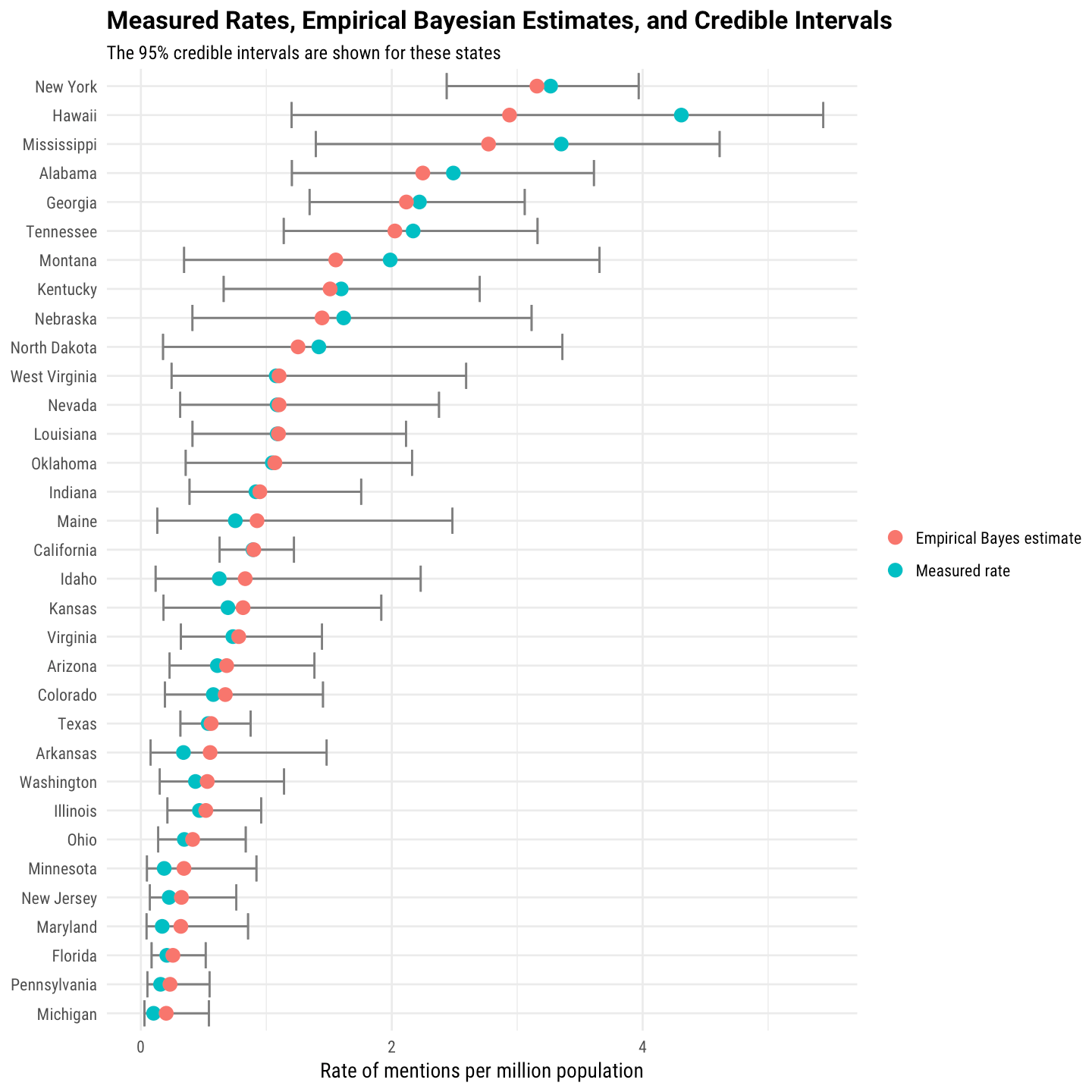## The End

The part of this that was the most satisfying was the credible intervals, how big/small they are, and how the plain vanilla rates and empirical Bayes rate estimates are distributed in the credible intervals. It definitely gave me the data intuition warm fuzzies and made a lot of sense. This method is not that hard to implement or understand and was a gratifyingly productive first foray into Bayesian methods. The R Markdown file used to make this blog post is available here. I am very happy to hear feedback or questions!